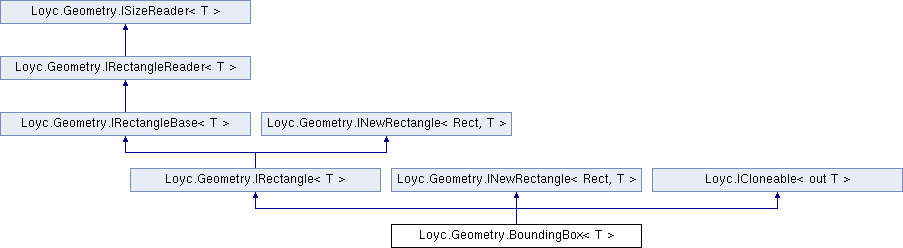Enhanced C# Language of your choice: library documentation

# Documentation moved to ecsharp.net

GitHub doesn't support HTTP redirects, so you'll be redirected in 3 seconds.

Loyc.Geometry.BoundingBox< T > Class Template Reference

Holds a mutable 2D bounding rectangle. More...

Source file:
Inheritance diagram for Loyc.Geometry.BoundingBox< T >:## Remarks

Holds a mutable 2D bounding rectangle.

Template Parameters
 T Data type of each coordinate.

Many extension methods are provided in BoundingBoxExt. They are separate from the class itself so that specialized versions are available for particular types (notably int, double and float).

X1 and Y1 should contains the minimum coordinates, while X2 and Y2 should contain the maximum coordinates. BoundingBox generally does not guarantee that this is true unless you call BoundingBoxExt.Normalize{T}, or if you use a constructor that accepts two points (rather than explicit minimum and maximum coordinates.) Some methods will not work correctly if the "maximum" coordinate is less than the "minimum" coordinate; they may simply assume that the maximum exceeds the minumum.

A BoundingBox is considered to include both the minimum and maximum coordinates. A point on the border is considered to be within the bounding box, and the Width and Height are incremented to account for this. For example, if T is int and (X1,X2)=(0,10), the Width is 11; if T is float and (X1,X2)=(0,10), the Width is infitessimally more than 10 (the result is incremented with MathEx.NextHigher()).

Type Constraints
 T : IConvertible T : IComparable T : IEquatable

## Properties

X1` [get, set]`

Y1` [get, set]`

X2` [get, set]`

Y2` [get, set]`

Width` [get, set]`

Height` [get, set]`

Point< T > MinPoint` [get, set]`

Point< T > MaxPoint` [get, set]`Properties inherited from Loyc.Geometry.IRectangleReader< T >
X1` [get]`

Y1` [get]`

X2` [get]`

Y2` [get]`Properties inherited from Loyc.Geometry.ISizeReader< T >
Width` [get]`
Gets the width of a rectangle (the difference between X coordinates). More...

Height` [get]`
Gets the height of a rectangle (the difference between Y coordinates). More...

## Public Member Functions

BoundingBox (T minX, T minY, T maxX, T maxY)

BoundingBox (Point< T > p)

BoundingBox (Point< T > p1, Point< T > p2)

BoundingBox< T > NewRect (T x, T y, T width, T height)

BoundingBox< T > NewRange (T x1, T y1, T x2, T y2)

void SetXAndWidth (T x, T width)

void SetYAndHeight (T y, T height)

void SetXRange (T x1, T x2)

void SetYRange (T y1, T y2)

bool IsNormal ()

void SetRect (T x, T y, T width, T height)

void SetRange (T x1, T y1, T x2, T y2)

BoundingBox< T > Clone ()

override string ToString ()